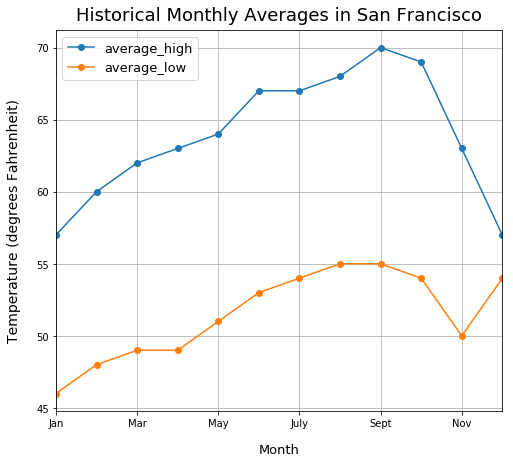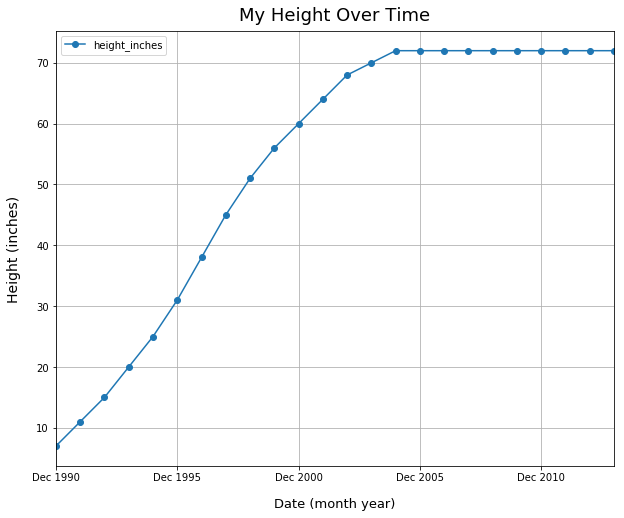Data Visualizations Best Practices Tutorial

# When to Use Line Plots

Line plots are often best to illustrate the change in a variable over time.

Unlike bar plots, I think the variable in line plots should not be a sum or count metric. For example, I would use a line plot for visualizing temperature data over time because temperature is just a single recorded value.

### Import Modules¶

In :
import pandas as pd
import matplotlib.pyplot as plt
%matplotlib inline


### Example: Historic Weather Monthly Averages in San Francisco¶

I want to see the general trend of the change in temperature for the months in San Francisco, California.

#### Record Weather Data in Python Lists¶

In :
average_lows = [46, 48, 49, 49, 51, 53, 54, 55, 55, 54, 50, 54]
average_highs = [57, 60, 62, 63, 64, 67, 67, 68, 70, 69, 63, 57]

In :
months = [date.strftime("%b") for date in pd.date_range(start='1/2017', end='1/2018', freq='M')]


#### Plot Monthly Weather Data¶

In :
df = pd.DataFrame({'month': months, 'average_high': average_highs,
'average_low': average_lows})

In :
df.set_index('month').plot(kind='line', figsize=(8, 7), grid=True, style='o-')
plt.title("Historical Monthly Averages in San Francisco", fontsize=18, y=1.01)
plt.legend(fontsize=13);#### Explanation of Historical Monthly Averages Plot¶

The coolest months in San Francisco tend to be December to February while the warmest ones tend to be June to October.

### Example: Change in Height Over Time¶

As a child, when I did yearly checkups at my doctor's office, my doctor recorded my height. Below, I plotted fictional data of my height over time.

#### Create Data for Month-Year Values and Height¶

In :
month_years = [date.strftime("%b %Y") for date in pd.date_range(start='12/30/1990', end='12/31/2013', freq='A')]

In :
heights_inches = [7, 11, 15, 20, 25, 31, 38, 45, 51, 56, 60, 64, 68, 70, 72, 72, 72, 72, 72, 72, 72, 72, 72, 72]


#### Plot Change in Height Over Time¶

In :
df2 = pd.DataFrame({'month_year': month_years, 'height_inches': heights_inches})

In :
df2.set_index('month_year').plot(kind='line', figsize=(10, 8), grid=True, style='o-')
plt.title("My Height Over Time", fontsize=18, y=1.01)